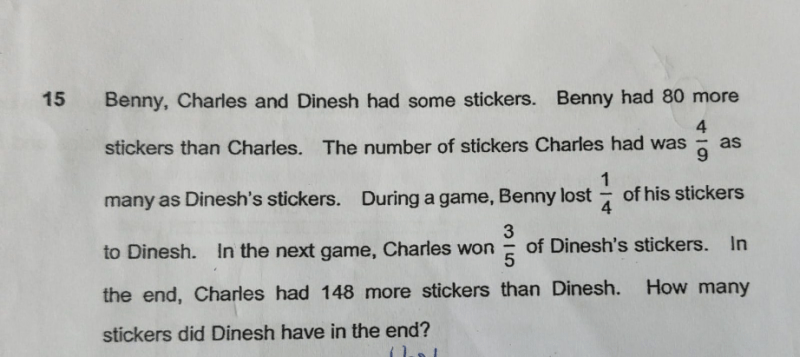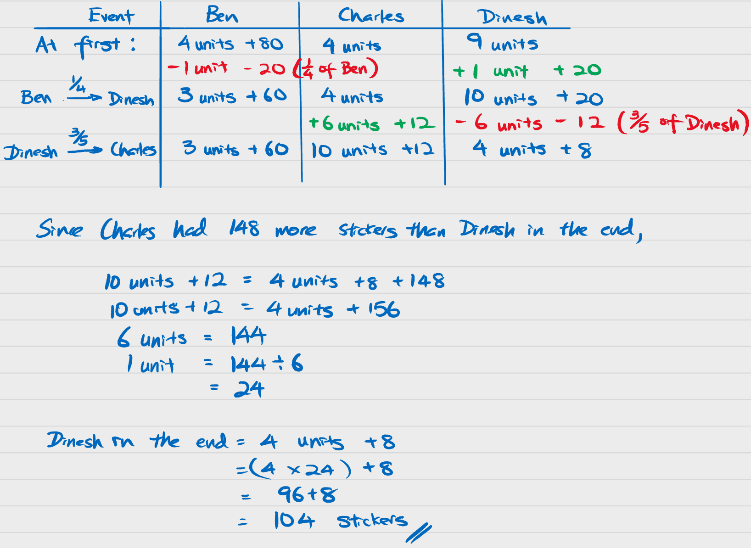# QuestionI am glad I have found your post because I am also searching for this solution online. I am a college student and I want to solve this equation but I can’t solve it. Thank you so much for sharing this with us. Do you like to play real money games and if yes then you should see this in which you find a lot of real money gaming sites. I also play over there so that I can pay my college fees.

0 Replies 0 Likes

Let x be the number of stickers Charles has.

So Benny has x+80 stickers, and Dinesh has (9/4)*x stickers

During the game, Benny lost 1/4 of his stickers, so he is left with (3/4)x + 60, and Dinesh gets the same amount so Dinesh has (10/4)x + 20 stickers.

In the next game, Charles wins 3/5 of Dinesh’s stickers, which is 3/5 * [(10/4) * x + 20]= (3/2)* x + 12

So Charles now has x + (3/2)x  + 12 = (5/2)x + 12 stickers, while Dinesh is left with (2/5)*[(10/4)x+20] = x + 8 stickers

So (5/2)x + 12 = 148 + (x + 8)

(3/2)x = 144

x = 144 * 2/3 = 96

Dinesh would have 96 + 8 = 104 stickers.